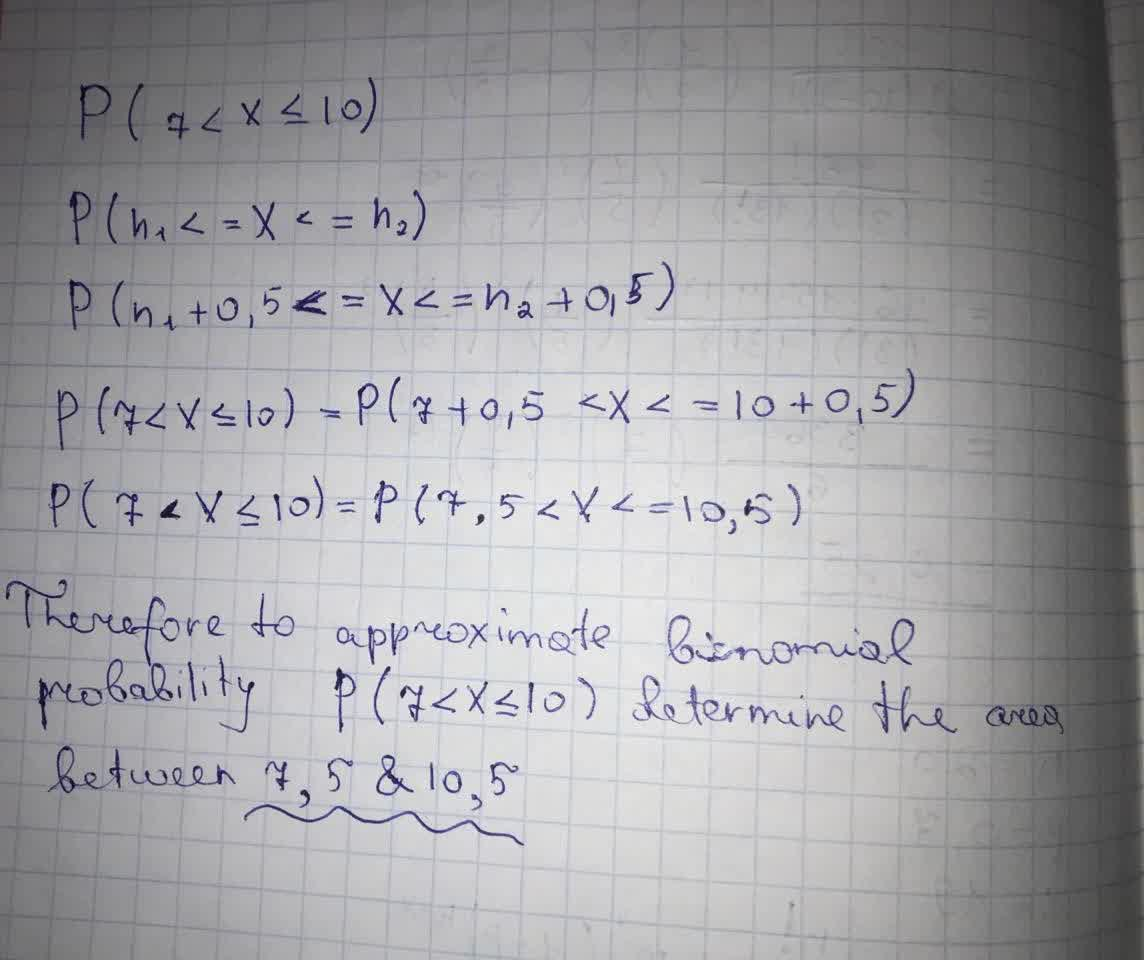# For each exercise, indicate which area under the appropriate normal curve would be determined to approximate the specified binomial probability.Khaleesi Herbert 2021-09-08 Answered

X denotes a binomial random variable with parameters n and p. For each exercise, indicate which area under the appropriate normal curve would be determined to approximate the specified binomial probability. $P\left(7

You can still ask an expert for help

• Questions are typically answered in as fast as 30 minutes

Solve your problem for the price of one coffee

• Math expert for every subject
• Pay only if we can solve itViktor Wiley